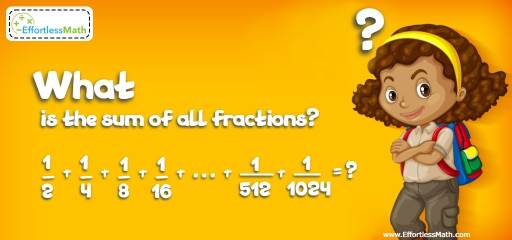# Intelligent Math Puzzle – Challenge 84

This math challenge requires basic math knowledge and some kind of logic and creative thinking to solve. Let's challenge your brain!## Challenge:

What is the sum of all fractions?
$$\frac{1}{2} + \frac{1}{4} + \frac{1}{8} + \frac{1}{16} + … + \frac{1}{512} + \frac{1}{1024} =$$

### The Absolute Best Book to Challenge Your Smart Student!

The correct answer is $$\frac{1023}{1024}$$.

$$\frac{1}{2} + \frac{1}{4} + \frac{1}{8} + \frac{1}{16} + … + \frac{1}{512} + \frac{1}{1024} =$$
To add the fractions, we need to find the common denominator.
The common denominator is 1024. Therefore:
$$\frac{512}{1024} +\frac{ 256}{1024} + \frac{128}{1024} + … + \frac{2}{1024} + \frac{1}{1024} =$$
$$\frac{512 + 256 + 128 + 64 + 32 + 16 + 8 + 4 + 2 + 1 }{1024} =\frac{1023}{1024}$$

The Best Books to Ace Algebra

### What people say about "Intelligent Math Puzzle – Challenge 84 - Effortless Math: We Help Students Learn to LOVE Mathematics"?

No one replied yet.

X
45% OFF

Limited time only!

Save Over 45%

SAVE $40 It was$89.99 now it is \$49.99